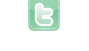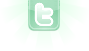## how to find nearest object.

so dan with the auto fire it works but not as intendid so it does fire out but it fires out like 2-3 strands of bullets at the same time and i dont want that and it also didnt turn the player to face where it was shooting. so i figured that i would have to use the getobjects in range function but i cant figure out how to do it here is the code:
```if(ammo>0){
if(!getObjectsInRange(350, Zombie.class).isEmpty()){
int dist=350;

while(!getObjectsInRange(dist, Zombie.class).isEmpty()){
dist--;
}
dist++;

Actor target = (Zombie) getObjectsInRange(dist,Zombie.class).get(0);

Actor bullet = new bullet();
bullet.turnTowards(target.getX(), target.getY());
}
}```
envxity wrote...
so dan with the auto fire it works but not as intendid so it does fire out but it fires out like 2-3 strands of bullets at the same time and i dont want that and it also didnt turn the player to face where it was shooting. so i figured that i would have to use the getobjects in range function but i cant figure out how to do it here is the code: << Code Omitted >>
Try this:
```if (ammo > 0) {
if (getWorld().getObjects(Zombie.class).isEmpty()) return;
Actor closest = null;
int closeness = 9999;
for (Object obj : getWorld().getObjects(Zombie.class)) {
Actor enemy = (Actor)obj;
int dist = (int)Math.hypot(getX()-enemy.getX(), getY()-enemy.getY());
if (dist < closeness) {
closeness = dist;
closest = enemy;
}
}
Actor bullet = new bullet();